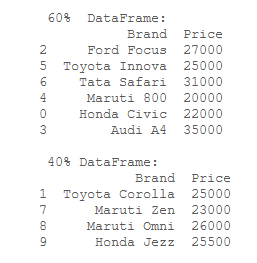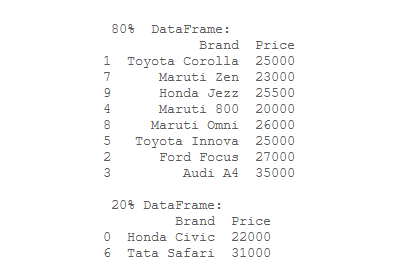# Divide a DataFrame in a ratio

• Last Updated : 17 Aug, 2020

Pandas is an open-source library that is built on top of numpy library. A Dataframe is a two-dimensional data structure, like data is aligned in a tabular fashion in rows and columns. DataFrame.sample() Method can be used to divide the Dataframe.

Syntax: DataFrame.sample(n=None, frac=None, replace=False, weights=None, random_state=None, axis=None)

frac attribute is the one which defines the fraction of Dataframe to be used. For example frac = 0.25 indicates that 25% of the Dataframe will be used.

Now, Let’s create a Dataframe:

## Python3

 `# importing pandas as pd ``import` `pandas as pd`` ` `# dictionary``cars ``=` `{``  ``'Brand'``: [``'Honda Civic'``, ``'Toyota Corolla'``, ``            ``'Ford Focus'``, ``'Audi A4'``, ``'Maruti 800'``,``            ``'Toyota Innova'``, ``'Tata Safari'``, ``'Maruti Zen'``, ``            ``'Maruti Omni'``, ``'Honda Jezz'``],``   ``'Price'``: [``22000``, ``25000``, ``27000``, ``35000``,``             ``20000``, ``25000``, ``31000``, ``23000``,``             ``26000``, ``25500``]`` ``}`` ` `# create the dataframe ``df ``=` `pd.DataFrame(cars, ``                  ``columns ``=` `[``'Brand'``,``                             ``'Price'``])``# show the dataframe``df`

Output:Example 1: Divide a given Dataframe in 60% and 40%.

## Python3

 `# importing pandas as pd ``import` `pandas as pd`` ` `# dictionary``cars ``=` `{``  ``'Brand'``: [``'Honda Civic'``, ``'Toyota Corolla'``, ``            ``'Ford Focus'``, ``'Audi A4'``, ``'Maruti 800'``,``            ``'Toyota Innova'``, ``'Tata Safari'``, ``'Maruti Zen'``, ``            ``'Maruti Omni'``, ``'Honda Jezz'``],``   ``'Price'``: [``22000``, ``25000``, ``27000``, ``35000``,``             ``20000``, ``25000``, ``31000``, ``23000``,``             ``26000``, ``25500``]`` ``}`` ` `# create the dataframe ``df ``=` `pd.DataFrame(cars, ``                  ``columns ``=` `[``'Brand'``,``                             ``'Price'``])`` ` `# Print the 60% of the dataframe ``part_60 ``=` `df.sample(frac ``=` `0.6``)``print``(``"\n 60%  DataFrame:"``)``print``(part_60)`` ` `# Print the 40% of the dataframe ``part_40 ``=` `df.drop(part_60.index)``print``(``"\n 40% DataFrame:"``)``print``(part_40)`

Output:Example 2: Divide a given Dataframe in 80% and 20%.

## Python3

 `# importing pandas as pd ``import` `pandas as pd`` ` `# dictionary``cars ``=` `{``  ``'Brand'``: [``'Honda Civic'``, ``'Toyota Corolla'``, ``            ``'Ford Focus'``, ``'Audi A4'``, ``'Maruti 800'``,``            ``'Toyota Innova'``, ``'Tata Safari'``, ``'Maruti Zen'``, ``            ``'Maruti Omni'``, ``'Honda Jezz'``],``   ``'Price'``: [``22000``, ``25000``, ``27000``, ``35000``,``             ``20000``, ``25000``, ``31000``, ``23000``,``             ``26000``, ``25500``]`` ``}`` ` `# create the dataframe ``df ``=` `pd.DataFrame(cars, ``                  ``columns ``=` `[``'Brand'``,``                             ``'Price'``])`` ` `# Print the 80% of the dataframe ``part_80 ``=` `df.sample(frac ``=` `0.8``)``print``(``"\n 80%  DataFrame:"``)``print``(part_80)`` ` `# Print the 20% of the dataframe ``part_20 ``=` `df.drop(part_80.index)``print``(``"\n 20% DataFrame:"``)``print``(part_20)`

Output:My Personal Notes arrow_drop_up